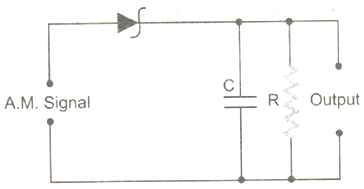# Communications Systems

15 QuestionsSettingsTime: 30 Minute

Related Topics
• 1.
The audio frequencies range from:
• A.

20 Hz to 20,000 kHz

• B.

20 Hz to 20 kHz

• C.

20 kHz to 20,.000 kHz

• D.

None of these

• 2.
The length of a half wave dipole at 30 mega-hertz is:
• A.

5 m

• B.

10 m

• C.

25 m

• D.

80 m

• 3.
A TV station transmits signals of 500 MHz. What is the wavelength used by the TV station?
• A.

0.3 m

• B.

0.6 m

• C.

0.6 km

• D.

None of these

• 4.
A radio can tune to any station in the frequency range of7.5 MHz to 12 MHz band. The corresponding wavelength band is:
• A.

40 m to 20 m

• B.

80 m to 40 m

• C.

40 m to 25 m

• D.

80 m to 60 m

• 5.
Calculate the phase velocity of electromagnetic wave having electron density and frequency for D layer, N = 40 electron/cc, v = 300 kHz:
• A.
• B.
• C.
• D.
• 6.
When radio waves passes through ionosphere, phase difference between space current and capacitive displacement current is:
• A.

• B.

• C.

• D.

• 7.
Which of the following frequencies will be suitable far beyond the horizon communication?
• A.

10 kHz

• B.

10 MHz

• C.

1 GHz

• D.

1000 GHz

• 8.
Ozone layer above Earth’s atmosphere will:
• A.

Prevent infrared radiations from sun reaching Earth.

• B.

Prevent infrared rays reflected from Earth from escaping Earth’s atmosphere.

• C.

Prevent ultraviolet rays from sun.

• D.

• 9.
Arrange the following communication frequency bands in the increasing order of frequencies: 1. AM Broadcast 2. Cellular mobile radio 3. FM Broadcast 4. Television UHF 5. Satellite communication
• A.

1, 3, 4, 2, 5

• B.

1, 2, 3, 4, 5

• C.

5, 2, 4, 3, 1

• D.

1, 3, 2, 4, 5

• 10.
A radio-station has two channels. One is AM at 1020 kHz and other FM at 89.5 MHz. For good results you will use:
• A.

Longer antenna for the AM channel and shorter for the FM

• B.

Shorter antenna for the AM channel and longer for the FM

• C.

Same length antenna will work for both

• D.

Information given is not enough to say which one to use for which

• 11.
The sky wve propagation is suitable for radio-waves of frequency:
• A.

Upto 2 MHz

• B.

From 2 MHz to 20 MHz

• C.

From 2 MHz to 30 MHz

• D.

From 2 MHz to 50 MHz

• 12.
Given below is the circuit diagram of an AM demodulator. For good demodulation of AM signal of carrier frequency f, the value of RC should be:
• A.

RC = 1/f

• B.

RC < 1/f

• C.

RC ≤ 1/f

• D.

RC >> 1/f

• 13.
A transparent solid cylindrical rod has a refractive index of  . It is surrounded by air. A light ray is incident at the mid–point of one end of the rod as shown in the figure. The incident angle θ for which the light ray grazes along the wall of the rod is:
• A.
• B.
• C.
• D.
• 14.
If both the lens of an antenna and the wavelength of the signal to be transmitted are doubled, the power radiated by the antenna:
• A.

Is doubled

• B.

Is halved

• C.

Increases 16 times

• D.

Remains constant

• 15.
A radar has a power of 1KW and is operating at a frequency of 10 GHz. It is located on a mountain top of height 500 m. The maximum distance upto which it can detect object located on the surface of the earth (Radius of the Earth = 6.4 X 106 m) is:
• A.

80 km

• B.

64 km

• C.

40 km

• D.

16 km